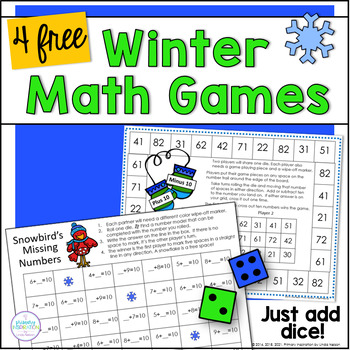# Winter Math Center Games - Addition, Subtraction, and Place Value1st - 2nd, Homeschool
Subjects
Standards
Resource Type
Formats Included
• PDF
•Activity
Pages
4 student pages
Easel Activity Included
This resource includes a ready-to-use interactive activity students can complete on any device. Easel by TpT is free to use! Learn more.

#### Also included in

1. Is your class ready for snowy days? Here's a collection of themed addition, subtraction, missing addend, missing subtrahend, and place value activities that you'll love ... and so will your students! This bundle will give you everything you need for your math centers all winter long!Important Note:
\$39.48
\$70.50
Save \$31.02
2. Here's a set of 80 low-prep winter math games for addition, subtraction, place value, and mixed practice, with enough variety and quantity to keep your math centers filled all winter long!This bundle includes four sets of partner games for first grade addition, subtraction, place value, and mixed pr
\$20.48
\$32.00
Save \$11.52

### Description

Are you looking for easy prep math games that will bring review topics into your math centers all winter? Try this set of four winter math games for addition, subtraction, place value, and missing addends! You'll find the variety you need for effective review!

Each game is just one page. There are no cards to print and cut ... which means that there are no cards to lose! Just add dice and you're ready to go! These games are so easy to prepare that you just might find yourself using them the same day that you download them!

Now includes a ready-to-use Easel version of each game! Whether you need print or digital, these games are ready for you!

These games are samples from Winter Math Games First Grade Bundle, 80 games for your winter math centers!

How can you use these games with your students?

* Math tubs or morning tubs

* Math centers

* Math intervention or RtI

* Home schooling

* Tutors

* Fun Friday

* Family Math Night

*****

Do you love using math games with your class? Here are a few more that you'll love!

Add and Subtract Within 20 | Independent Math Games and Activities Bundle

Addition Subtraction Place Value Math Games Bundle

*****

Linda Nelson grants permission to the original downloader to reproduce material for his or her own classroom use only. No other part of this work may be reproduced in whole or in part by electronic or mechanical means including information storage and retrieval systems without permission in writing from the author.

*****

Click here to follow me. You’ll get an email update whenever I post a new resource. You’ll also find an occasional “Followers Only” freebie in your TpT inbox, just to say thanks!☺

Linda Nelson

Primary Inspiration by Linda Nelson

Total Pages
4 student pages
N/A
Teaching Duration
N/A
Report this Resource to TpT
Reported resources will be reviewed by our team. Report this resource to let us know if this resource violates TpT’s content guidelines.

### Standards

to see state-specific standards (only available in the US).
Determine the unknown whole number in an addition or subtraction equation relating three whole numbers. For example, determine the unknown number that makes the equation true in each of the equations 8 + ? = 11, 5 = ▯ - 3, 6 + 6 = ▯.
Add and subtract within 20, demonstrating fluency for addition and subtraction within 10. Use strategies such as counting on; making ten (e.g., 8 + 6 = 8 + 2 + 4 = 10 + 4 = 14); decomposing a number leading to a ten (e.g., 13 - 4 = 13 - 3 - 1 = 10 - 1 = 9); using the relationship between addition and subtraction (e.g., knowing that 8 + 4 = 12, one knows 12 - 8 = 4); and creating equivalent but easier or known sums (e.g., adding 6 + 7 by creating the known equivalent 6 + 6 + 1 = 12 + 1 = 13).
Understand subtraction as an unknown-addend problem. For example, subtract 10 – 8 by finding the number that makes 10 when added to 8.
Apply properties of operations as strategies to add and subtract. If 8 + 3 = 11 is known, then 3 + 8 = 11 is also known. (Commutative property of addition.) To add 2 + 6 + 4, the second two numbers can be added to make a ten, so 2 + 6 + 4 = 2 + 10 = 12. (Associative property of addition.)
Given a two-digit number, mentally find 10 more or 10 less than the number, without having to count; explain the reasoning used.